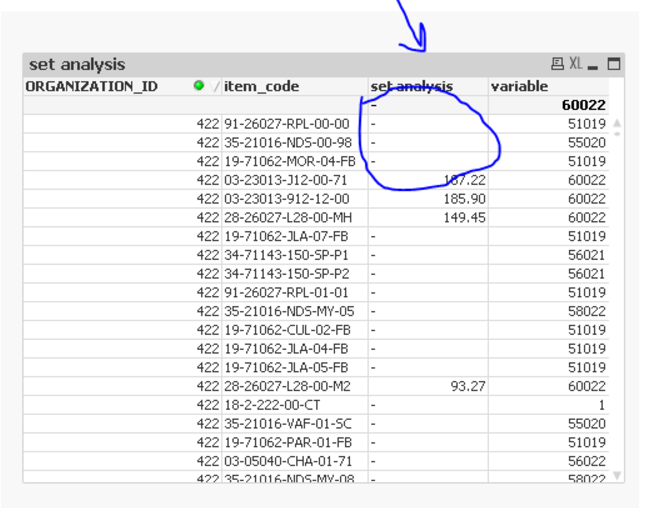# New to QlikView

Discussion board where members can get started with QlikView.

Announcements
Save the Date: QlikWorld Online, June 24-25, 2020. Free global virtual event for data integration and data analytic gurus. Register Today
Contributor

## variable evaluation in set analysis

hey all

I have created a variable as follows

vMax_Period_ID

max({<MonthYR={"<=\$(=max(AWESOME_IDEA_2))"}>}PAC_PERIOD_ID) " no equal sign prior to formula"

now im trying to use this variable in a set analysis and this variable should return one value for each item

my set analysis

=only({<PAC_PERIOD_ID={"\$(=\$(vMax_Period_ID))"}>}ITEM_COST)

yet the variable doesn't evaluate properly

if we replace the variable with a hard coded value it works just fine1 Solution

Accepted Solutions
HighlightedMVP

## Re: variable evaluation in set analysis

I think I understand your issue.... if you look at the image you posted, only where variable is 60022, set analysis column has a value.The problem is that you are expecting set analysis to be evaluated for each dimension, but it only evaluates per chart. So in your case the Max({<MonthYR={"<=\$(=Max(AWESOME_IDEA_2))"}>}PAC_PERIOD_ID) = 60022 which is used across all your dimensions. In order to resolve this issue.... you can use Aggr() function instead of using set analysis.

Aggr(If(PAC_PERIOD_ID = Max(TOTAL <ORGANIZATION_ID, item_code> {<MonthYR={"<=\$(=Max(AWESOME_IDEA_2))"}>}PAC_PERIOD_ID), ITEM_COST), ORGANIZATION_ID, item_code, PAC_PERIOD_ID)

8 Replies
Highlighted
Honored Contributor

## Re: variable evaluation in set analysis

Hi,

Try

Aggr(=only({<PAC_PERIOD_ID={"\$(=\$(vMax_Period_ID))"}>}ITEM_COST), ITEM_COST)

HighlightedMVP

## Re: variable evaluation in set analysis

Or, may be even without aggr(), like:

only({<PAC_PERIOD_ID={"\$(=\$(vMax_Period_ID))"}>}ITEM_COST)

Highlighted
Contributor

## Re: variable evaluation in set analysis

Thank you guys but your responses have not changed anything

HighlightedMVP

## Re: variable evaluation in set analysis

What if you replace the variable's double quotes to single quote

Max({<MonthYR={'<=\$(=Max(AWESOME_IDEA_2))'}>}PAC_PERIOD_ID)

Highlighted
Contributor

## Re: variable evaluation in set analysis

the variable actually evaluates correctly but not when inserted  into set analysis

if you may see my above picture ,the variable column actually returns corrects values

im assuming that the variable is not evaluated properly in the set analysis could be syntax 'single or double quotation, dollar sign expansion '

HighlightedMVP

## Re: variable evaluation in set analysis

I think I understand your issue.... if you look at the image you posted, only where variable is 60022, set analysis column has a value.The problem is that you are expecting set analysis to be evaluated for each dimension, but it only evaluates per chart. So in your case the Max({<MonthYR={"<=\$(=Max(AWESOME_IDEA_2))"}>}PAC_PERIOD_ID) = 60022 which is used across all your dimensions. In order to resolve this issue.... you can use Aggr() function instead of using set analysis.

Aggr(If(PAC_PERIOD_ID = Max(TOTAL <ORGANIZATION_ID, item_code> {<MonthYR={"<=\$(=Max(AWESOME_IDEA_2))"}>}PAC_PERIOD_ID), ITEM_COST), ORGANIZATION_ID, item_code, PAC_PERIOD_ID)

Highlighted
Contributor

## Re: variable evaluation in set analysis

Thank you so you Much Sunny,

your answer is correct and it is giving me correct values yet Im missing the logic you used there ,what total has to do here ???

HighlightedMVP

## Re: variable evaluation in set analysis

Although your table has only two dimensions, I have using three dimensions in the Aggr() function. The reason I had to use PAC_PERIOD_ID as a dimension is because I am using that in the If statement non aggregated which requires me to add it as a dimension.

Aggr(If(PAC_PERIOD_ID = Max(TOTAL <ORGANIZATION_ID, item_code> {<MonthYR={"<=\$(=Max(AWESOME_IDEA_2))"}>}PAC_PERIOD_ID)

Now to get a single value (Max based on ORGANIZATION_ID and item_code), I had to use total key word with both the dimension.....

An easy way to see this would be to do like this Create a new straight table with three dimensions

ORGANIZATION_ID

item_code

PAC_PERIOD_ID

and try these expressions

1) PAC_PERIOD_ID

2) Max(TOTAL <ORGANIZATION_ID, item_code> {<MonthYR={"<=\$(=Max(AWESOME_IDEA_2))"}>}PAC_PERIOD_ID)

3) If(PAC_PERIOD_ID = Max(TOTAL <ORGANIZATION_ID, item_code> {<MonthYR={"<=\$(=Max(AWESOME_IDEA_2))"}>}PAC_PERIOD_ID), ITEM_COST)

Once you do this, you will sort of understand what I am doing here